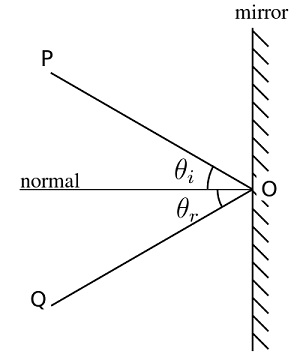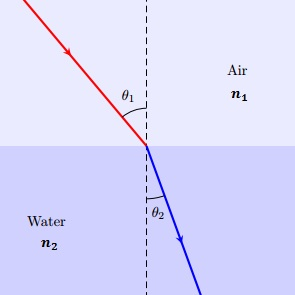# Difference Between Refraction and Reflection

## Main Difference – Reflection vs. Refraction

Reflection and refraction are two properties of waves that describe how a wave behaves when it meets a boundary between two media. The main difference between refraction and reflection is that reflection describes how a wave “rebounds” back towards the medium that it came from while refraction describes how a wave bends as it passes from one medium into the other. Reflection and refraction are properties exhibited by any type of wave. However, the discussion below will focus primarily on light waves.

## What is Reflection

When a wave meets a boundary between two media, a part of the wave returns back towards the medium that it came from. This phenomenon is referred to as reflection. If the wave is represented using a ray, we can depict reflection as follows:Law of Reflection

In the above diagram, the wave approaches from the top, so the line PO represents the incident ray. The normal is a line drawn perpendicular to the surface, through the point where the ray strikes the boundary. The angle$\theta_i$ is called the angle of incidence. The ray OQ is the reflected ray. The angle between the reflected ray and the normal is called the angle of reflection$\theta_r$.

The law of reflection states that the angle of incidence is equal to the angle of reflection. The incident ray, the reflected ray and the normal are all in one plane. Mirrors work by reflecting light that fall on it. In comparison, transparent glass reflects little of the incident light and lets most of it pass through.Reflections

## What is Refraction

Refraction is a phenomenon that happens when a wave goes from one medium into another. Here, the ray bends as it passes from one medium to the other. Absolute refractive index of a medium is a number that describes how much a ray of light would bend if the ray came from a vacuum and entered that medium. How the ray bends depends on the absolute refractive indices of the two media. If the ray goes from a medium with a lower absolute refractive index into a medium with a larger absolute refractive index, then the ray bends towards the normal. If the second medium has a lower refractive index than the first, then the ray bends away from the normal.Law of Refraction

In the above diagram,$n_1$ and$n_2$ refer to the absolute refractive indices of air and water respectively, and in this case$n_2>n_1$, and so the ray bends towards the normal. Precisely how much the ray bends is given by law of refraction, or Snell’s law. According to the law of refraction,$n_1\: \mathrm{sin}\: \theta_1=n_2\: \mathrm{sin}\: \theta_2$

Refraction is what makes objects seem “bent” when they are put into water. Refraction is also responsible for making swimming pools seem shallower they are because the light waves from the bottom of the pool bend as they go from the pool into the air. In microscopes and telescopes, we use the ability of lenses to bend light is used to produce magnified images of objects.Reflection and Refraction at a Lake

## Difference Between Refraction and Reflection

### How the wave travels

In Reflection, the wave turns back towards the medium that it originally came from.

In Refraction, the wave travels from one medium into another medium.

### Physical Principle

Reflection is described by the law of reflection. This does not depend on the refractive indices between the media.

Refraction is described by Snell’s law: the ratio of the sines of the angles of incidence and refraction is proportional to the ratio of absolute refractive indices of the two media.

### Where they are used

Reflection is utilized in mirrors.

Refraction is utilized by lenses.

Image Courtesy

“Angle of incidence equals the angle of reflection on a mirror.” by Johan Arvelius (Own work) [CC BY-SA 3.0], via Wikimedia Commons

“Reflections” by Beverley Goodwin (Own work) [CC BY-SA 2.0], via flickr

“Example: Refraction – Snell’s Law” by Jimi Oke (Own work) [CC BY 2.5], via TEXample.net (Modified)

“Refraction and Reflection” by David Dixon (Own work) [Copyrighted, licenced for reuse under CC BY-SA 2.0], via Geograph